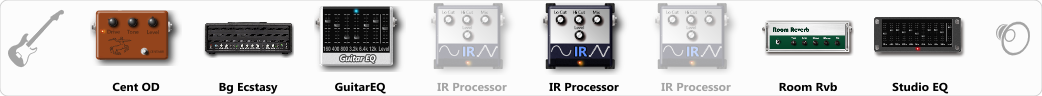# Big Ecs Ed VH Cabs

Discussion in 'ToneLib-GFX presets' started by NickyFingers, Jan 6, 2020.

1. Big Ecs Ed VH Cabs

Preset name: Big Ecs Ed VH Cabs

Effects chain:Effect: "Cent OD" (Overdrive / Distortion), active - "yes"
"Drive" = 38
"Tone" = 62
"Level" = 75

Effect: "Bg Ecstasy" (Amp simulators), active - "yes"
"Gain" = 76
"Bass" = 45
"Middle" = 50
"Treble" = 65
"Presence" = 56
"Master" = 50
"Output" = 50
"Level (dB)" = 0

Effect: "GuitarEQ" (Dynamics / Filter), active - "yes"
"160 Hz" = -5
"400 Hz" = -3
"800 Hz" = -1
"1.6 kHz" = 0
"3.2 kHz" = 0
"6.4 kHz" = 0
"12 kHz" = 0
"Level (dB)" = 0

Effect: "IR Processor" (Dynamics / Filter), active - "no"
"IR" = Ed VH High Off Axis
"Low Cut (Hz)" = 80
"Hi Cut (kHz)" = 20.0
"Mix" = 100
"Level (dB)" = 0

Effect: "IR Processor" (Dynamics / Filter), active - "yes"
"IR" = Ed VH Off Center Cap
"Low Cut (Hz)" = 80
"Hi Cut (kHz)" = 20.0
"Mix" = 100
"Level (dB)" = 0

Effect: "IR Processor" (Dynamics / Filter), active - "no"
"IR" = Ed VH Cap Edge
"Low Cut (Hz)" = 80
"Hi Cut (kHz)" = 20.0
"Mix" = 100
"Level (dB)" = 0

Effect: "Room Rvb" (Reverb), active - "yes"
"Time" = 4.5
"PreDelay" = 0
"LoDamp" = 46
"HiDamp" = 25
"Mix" = 41

Effect: "Studio EQ" (Dynamics / Filter), active - "yes"
"31 Hz" = 0
"62 Hz" = 0
"125 Hz" = -2
"250 Hz" = -8
"500 Hz" = -4
"1 kHz" = 0
"2 kHz" = 0
"4 kHz" = 0
"8 kHz" = 1
"16 kHz" = 0
"above 16 kHz" = 0
"Level (dB)" = 0

Note: You will need to download and install the ToneLib-GFX software to use the preset.

#### Attached Files:

• ###### Big_Ecs_Ed_VH_Cabs.tlgfx
File size:
36.5 KB
Views:
3,367
Leon1148, Gilly and Oscarsitonomas like this.